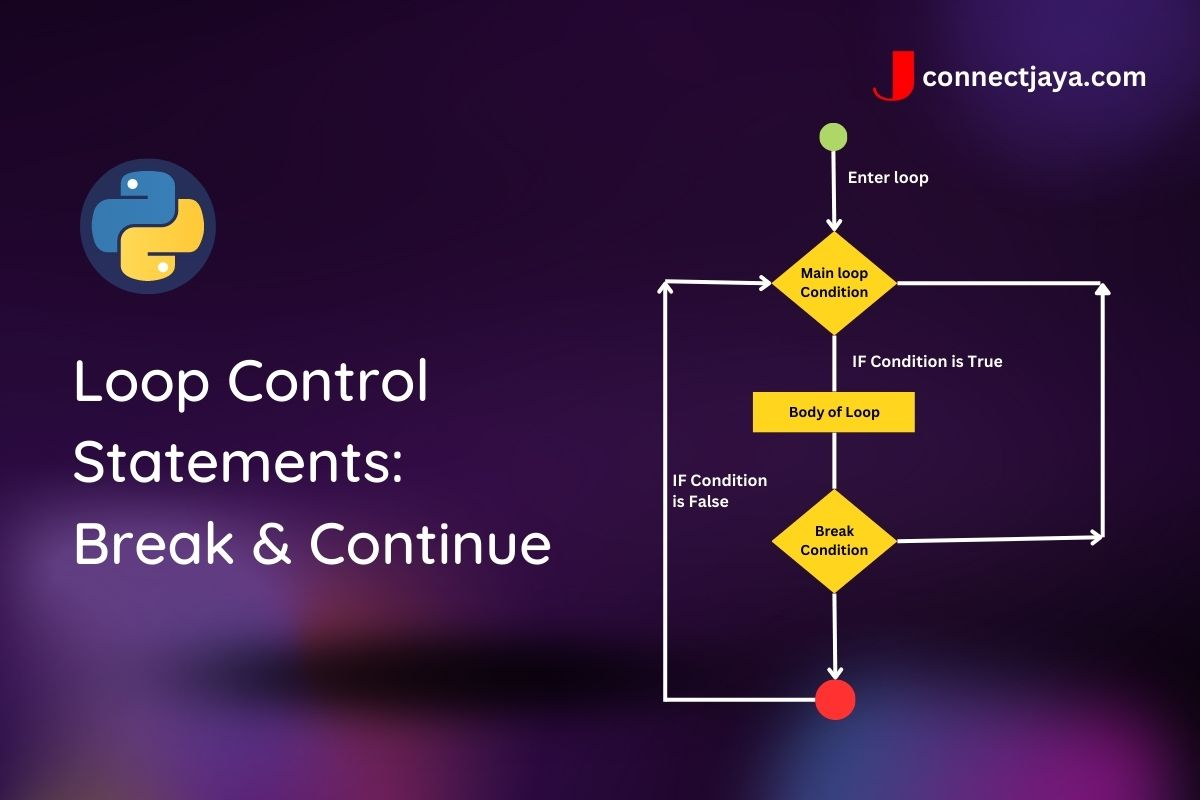## Loop Control Statements: Break & Continue

# Print all numbers from 1 to 10, but stop when you reach 7
for i in range(1, 11):
if i == 7:
break
print(i)


In this example, the program uses a for loop to iterate over the numbers from 1 to 10 using the range() function. Inside the loop, the program uses a conditional statement to check whether the current number is equal to 7 (if i == 7). If it is, the program exits the loop using the break statement. Otherwise, the program prints the current number using the print() function.

The output of this program would be:

1
2
3
4
5
6


Notice that the program stops when it reaches 7, because the break statement causes the loop to exit early.

Another loop control statement you can use is continue. Here’s an example:

# Print all odd numbers from 1 to 10, but skip over the even numbers
for i in range(1, 11):
if i % 2 == 0:
continue
print(i)


In this example, the program uses a for loop to iterate over the numbers from 1 to 10 using the range() function. Inside the loop, the program uses a conditional statement to check whether the current number is even (if i % 2 == 0). If it is, the program skips over that number using the continue statement. Otherwise, the program prints the current number using the print() function.

The output of this program would be:

1
3
5
7
9


Notice that the program skips over the even numbers (2, 4, 6, and 8) because of the continue statement.

I hope this example helps, but let me know if you have any other questions or if you’d like to see more examples!

# Print all even numbers from 1 to 10, but stop when you reach 6
for i in range(1, 11):
if i == 7:
break
if i % 2 != 0:
continue
print(i)


In this example, the program uses a for loop to iterate over the numbers from 1 to 10 using the range() function. Inside the loop, the program first checks if the current number is equal to 7 (if i == 7). If it is, the program exits the loop using the break statement.

Otherwise, if the current number is not even (if i % 2 != 0), the program skips over it using the continue statement. Otherwise, the program prints the current number using the print() function.

The output of this program would be:

2
4
6


Notice that the program stops when it reaches 6, because of the break statement. It also skips over the odd numbers using the continue statement.

I hope this example helps! Let me know if you have any other questions.